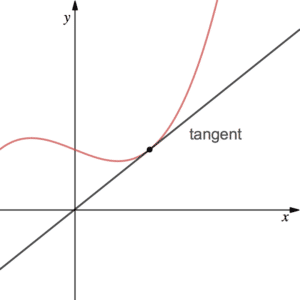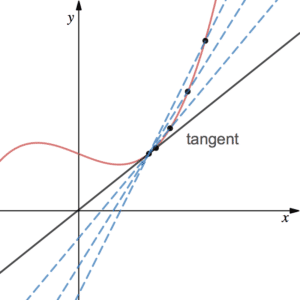# Differentiation from First Principles – gradient of a curve

Alongside integration, differentiation is the one of two main branches of calculus. We use it when finding the gradient of a curve as opposed to a straight line. It is derived from a method known as differentiation from first principles.Recall that the gradient of a straight line can be found by looking at its equation (the $m$ in $y=mx+c$). We find it by calculating rise over run. For a curve, however, the gradient is always changing. Thus we define the gradient of the curve at a given point to be the gradient of the tangent at that point (see more on tangents). The function that tells us the gradient of the tangent/curve at the given point is known as the derivative. Since the gradient is dependent on x, the derivative should also be a function of $x$. For $y=f(x)$ the derivative is often denoted $\frac{dy}{dx}$ or $f'(x)$. Note that we use the notation $\frac{dy}{dx}$ for rise over run on a curve. It denotes an infinitely small change in $x$ over an infinity small change in $y$. It represents the rate of change of $y$ with respect to $x$. Once an expression for the derivative is obtained, it can be used to calculate the gradient at any point. See Polynomial Examples below.

## Differentiation from First PrinciplesThe formal technique for finding the gradient of a tangent is known as Differentiation from First Principles. By taking two points on the curve that lie very closely together, the straight line between them will have approximately the same gradient as the tangent there. Evidently, the closer these points are together, the better the approximation.Suppose we want to find the gradient of the curve of $y=f(x)$ at a general point $(x,f(x))$. Choose another point close by $(x+h,f(x+h))$ where $h$ is small. Hence, rise over run is given by $\frac{f(x+h)-f(x)}{x+h-x}$.  By taking $h$ to be as small as possible, i.e. the limit as $h$ tends to 0 (what is a limit?), then rise over run will be the same as the gradient of the tangent. This is known as DIFFERENTIATION FROM FIRST PRINCIPLES:

$f'(x)=\lim_{h\rightarrow 0}\frac{f(x+h)-f(x)}{h}$

See Examples 1 and 2. Note that this is the formal approach for finding the derivative. Shortcuts are, however, usually adopted. See Polynomial Differentiation below.

Page 8 of the StudyWell Differentiation eGuide has some exam-style questions on differentiation from first principles to try:

## Differentiating Polynomials (a result from differentiation from first principles)

We can show by differentiating from first principles, that $\frac{d}{dx}\left(x^n\right)=nx^{n-1}$. For example, if $y=x^3$ then $\frac{dy}{dx}=3x^2$. It follows that the point (2,8) on the cubic graph has a gradient of 12. We can find this by putting $x=2$ into the derivative. We can then apply the principle to linear combinations of powers of $x$, also known as polynomials. For example, the polynomial $f(x)=5x^2-2x^4$ has the derivative $f'(x)=10x-8x^3$. We can think of it informally as ‘multiplying down by the power, then taking one off of the power’.

Note that, in maths, differentiation is finding the derivative or gradient function. The gradient of the straight line, $y=4x+3$, for example, is 4 – constants disappear. A question may expect you to simplify first. See Examples 3 to 5.

Page 12 of the StudyWell Differentiation eGuide has some exam-style questions on differentiating polynomials to try:

## Examples of Differentiation

Differentiate $y=x^2$ from first principles.

Differentiate $f(x)=2x^3$ from first principles.

Find $\frac{dy}{dx}$ if

$y=4x^{2}-5x^{-1}+7x-2$.

If $f(x)=8x^{-\frac{1}{2}}+3x^{\frac{1}{2}}$, find the gradient of the curve $y=f(x)$ at the point with $x$-coordinate 4.

Differentiate $h(x)=\frac{(2x^2-5)(x-2)}{\sqrt{x}}$.